### Home > PC > Chapter 9 > Lesson 9.3.2 > Problem9-112

9-112.
1. Given f(x) =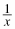, complete the following problems. Homework Help ✎

1. Compute the average rate of change from x = 2 to x = 3.

2. Show that the average rate of change from 2 to 2 + h is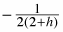.

3. Show that the average rate of change from x to x + h is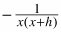.

4. What is the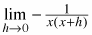?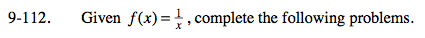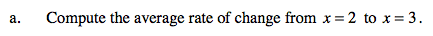$\frac{f(3)-f(2)}{3-2}$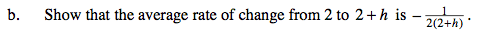$\frac{f(2+h)-f(2)}{2+h-2}$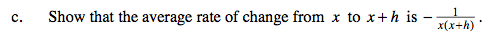$\frac{f(x+h)-f(x)}{x+h-x}$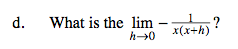Substitute 0 in for h.